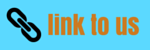# Class 10 Heating Effect of Electric current Notes

## 10. Heating Effect of Electric current

• When electric current passes through a high resistance wire, the wire becomes and produces heat. This is called heating effect of current.
• This phenomenon occurs because electrical energy is gets transformed into heat energy when current flows through a wire of some resistance say R Ω.
• Role of resistance in electrical circuits is similar to the role of friction in mechanics.
• To we will now derive the expression of heat produced when electric current flows through a wire.
• To we will now derive the expression of heat produced when electric current flows through a wire.
• For this consider a current I flowing through a resistor of resistance R. Let V be the potential difference across it as shown in the figure 6
• Let t be the time during which charge Q flows. Now when charge Q moves against the potential difference V , then the amount of work is given by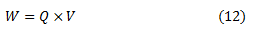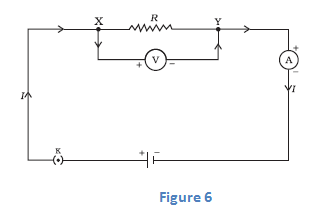Therefore the source must supply energy equal to VQ in time t. Hence power input to the circuit by the source is• The energy supplied to the circuit by the source in time t is P×t that is, VIt. This is the amount of energy dissipated in the resistor as heat energy.
• Thus for a steady current I flowing in the circuit for time t , the heat produced is given by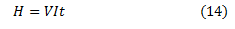Applying Ohm's law to above equation we get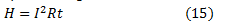This is known as Joule's Law of heating
• According to Joule's Law of Heating , Heat produced in a resistor is
(a) Directly proportional to the square of current for a given resistor.
(b) Directly proportional to resistance of a given resistor.
(c) Directly proportional to time for which current flows through the resistor.

## 11. Applications of heating effect of current

1. The heating effect of current is utilized in the electrical heating appliances for example electric iron, room heaters, water heaters etc.
2. The heating effect of electric current is utilized in electric bulbs or electric lamps for producing light.
3. An electric fuse is an important application of the heating effect of current. The working principle of a fuse wire is based on the heating effect of current. When high current flow through the fuse (current higher than the rated value) then the heat developed in the wire melts it and breaks the circuit.
4. In an electric heater one type of coil is used. A high resistance material like nichrome or same type of material is used as coil. The coil is wound in grooves on ceramic format or china clay. Flowing electric current through the coil it becomes heated. Due to high resistance the coil becomes red color forms.